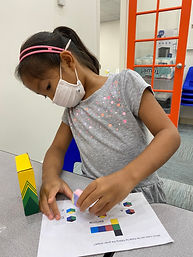Ms. Brianne

Target 1​

Lesson Type:

Continuation

Geometry

:

Symmetry

Understand that a figure does not change in a symmetrical reflection, the only change is positional.

1:

Relate a reflection to a mirror.

2:

Experiment with a mirror to see that a figure's reflection is backwards.

3:

Identify the reflection of a given image.

3rd

Vocabulary:

Symmetry, Symmetrical, Line of Symmetry, Reflection

Activities:

Students

• used pattern blocks to complete a symmetrical design when provided with one side of the design
• used mirrors to check for symmetry
• identified the reflection of a given shape
• drew the matching half of a shape or monster across a line of symmetryHome Exploration

Guiding Questions:Absent Students:

Target 2

:

1:

Complete a number sequence in both ascending and descending order.

2:

Start a number sequence from any given number.

Kindergarden

Vocabulary:

Ascending, Descending, Count on, Backwards

Activities:

Students

• practiced counting in both ascending and descending order
• wrote the numbers that come before or after a given numberHome Exploration

Guiding Questions:Target 3

:

1:

Think about objects in 3D and draw conclusions about them from limited information.

2:

Imagine an object being manipulated in space (rotated, folded, cut, etc.)

3:

Use spatial reasoning to solve problems.

1st

Vocabulary:

2D, 3D, Geometric net, Cube, Left, Right, Above, Below, Rotate, Flip

Activities:

Students

• observed a geometric net with various colors/shapes
• looked at a choice of cubes and determined the correct geometric cube that matched the geometric net
• checked their work by creating the geometric net shown and folding it into a cube to see if it matched the one they choseHome Exploration

Which cube can be made from folding the given geometric net?

Orange, Red, and Blue Cube